# Blog Archives

## NHS-R Community: Up the R-evolution

January 29, 2019
By

It seems like two seconds ago since I was frantically preparing slides for the first NHS community. Wow – what an experience and kudos to the team @NHS-R Community for arranging it. The full content of the event has been posted: https://nhsrcommunity.com/nhs-r-conference-9-october-2018/. What a fantastic event this was and I met many of my fellow...

## A simple function to create nice correlation plots

November 30, 2018
By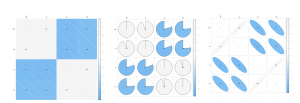The problem I was working with a dataset where I wanted to assess the correlation of different variables in R. As much as I like R – the outputs from the console window leave something to be desired (in terms of data visualisation). Therefore, I wanted a way to visualise these correlations in a nicer...

## A simple function to install and load packages in R

August 16, 2018
By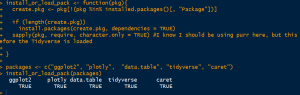One function to rule the world, or install or load your packages for you I was starting to despair at the amount of packages I end up using during the task of transforming, cleaning, modelling and validating some of my models. I thought there must be a simple approach to dealing with this? So, I...

## DPLYR: A Beginners Guide

May 24, 2018
By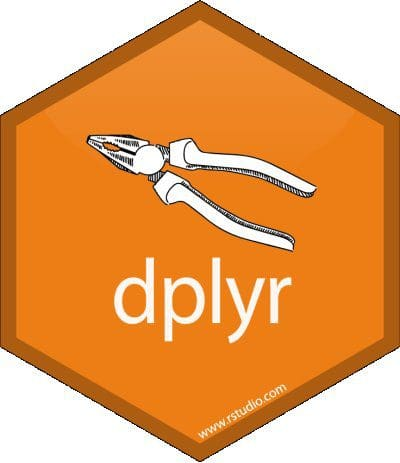What is DPLYR? Dplyr is a grammar of data manipulation, providing a consistent set of verbs that help you solve the most common data manipulation challenges. The next series of examples will show how you can use the shortcuts in Dplyr to achieve the results of traditional R data manipulation, but faster. Setting dplyr up...

## Violin and density plots in ggplot2

May 17, 2018
ByViolin Plots This chart is a combination of a Box Plot and a Density Plo that is rotated and placed on each side, to show the distribution shape of the data. The thick black bar in the centre represents the interquartile range, the thin black line extended from it represents the 95% confidence intervals, and the white dot is the...

## Box Plots in ggplot2

May 16, 2018
BySetting up the plot The box plot, in ggplot2, can be really powerful and useful for analysing variation. I will show a few examples of different types of box plots in ggplot2. Basic Box Plot library(ggplot2) theme_set(theme_classic()) # Plot plot

## Histogram with auto binning in ggplot2

May 16, 2018
By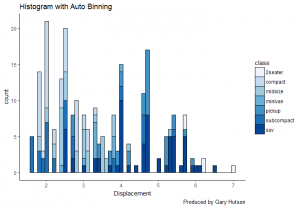Histograms (with auto binning) Again, we will use the mtcars dataset and use the fields in that to produce the chart, as we are doing this there is nothing to do on the data preparation side. That leaves us to have fun with the plot. Building the Histogram with auto binning I set up the...

## Diverging Dot Plot and Lollipop Charts – Plotting Variance with ggplot2

May 16, 2018
By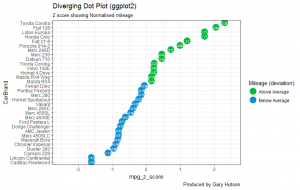Creating the Dot Plot Variance chart The data preparation was used in the previous blog entitled: Diverging Bar Charts – Plotting Variance with ggplot2. Refer to that if you need to know how to create the data prior to this tutorial. Setting up the Dot Plot Variance chart library(ggplot2) ggplot(mtcars, aes(x=CarBrand, y=mpg_z_score, label=mpg_z_score)) + geom_point(stat='identity', aes(col=mpg_type),...

## Diverging Bar Charts – Plotting Variance with ggplot2

May 16, 2018
By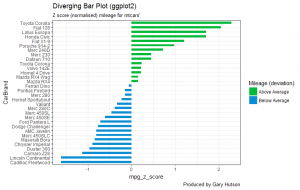Diverging Bar Charts The aim here is to create a diverging bar chart that shows variance above and below an average line. In this example I will use Z Scores to calculate the variance, in terms of standard deviations, as a diverging bar. This example will use the mtcars stock dataset, as most of the data I...